Pump

680 liters of water were pumped in 8 minutes. How many liters was spent in 56 minutes?

Result

V =  4760 l

Solution:Leave us a comment of this math problem and its solution (i.e. if it is still somewhat unclear...):Be the first to comment!To solve this verbal math problem are needed these knowledge from mathematics:

Tip: Our volume units converter will help you with the conversion of volume units.

Next similar math problems:

1. Comsumption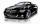On route 150 km long car consumed 13 liters of gasoline. How much gasoline consumed for driving 300 km?
2. Milk bill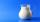Mrs Tara buys 2 liters of milk daily. If 1 liter of milk cost \$0.27. What will be her milk bill for 30 days.
3. HoursThe lesson lasts 45 minutes. For the week, students have 18 lesson hours. How many are the actual hours?
4. Hr to minSue biked to school in 5/12 of an hour. How many minutes did it take her to ride to school?
5. Pills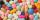If it takes 20 minutes to run a batch of 100 pills how many minutes would it take to run a batch of 50 pills
6. Minutes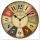Determine the difference in minutes: T1 = 2 3/20 h T2 = 2.3 h
7. Cows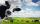4 cows spent 16 bags of hay in 5 days. How many bags of hay sacks is needed for 5 cows for seven days?
8. Working time - shortening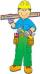The media often speculates about the change (especially to shorten) the working time of the five-day eight-hour working week to another working model. Calculate how many hours a day the employee would have to work a day at 3-day work week if he must work t
9. Steps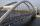Patrick step is 65 cm long and his dad step is 10 cm longer. Dad goes bridge in 52 steps. How many steps will go the same bridge by Patrick?
10. Father and son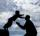Father is 44 years old, his son 16 years. Determine how many years ago was the father five times older than the son.
11. Chocolate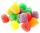I eat 24 chocolate in 10 days. How many chocolate I eat in 15 days at the same pace?
12. Math classificationIn 3A class are 27 students. One-third got a B in math and the rest got A. How many students received a B in math?
13. Rabbits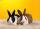Michael breeding rabbits. His 7 rabbits will eat 98 feed rations for 7 days. How many equal portions of feed should be eat 5 rabbits in 6 days?
14. Addition of Roman numbersAdded together and write as decimal number: LXVII + MLXIV
15. CagesHonza had three cages (black, silver, gold) and three animals (guinea pig, rat and puppy). There was one animal in each cage. The golden cage stood to the left of the black cage. The silver cage stood on the right of the guinea pig cage. The rat was in the
16. If-then equationIf 5x - 17 = -x + 7, then x =
17. Six te 2If 3t-7=5t, then 6t=